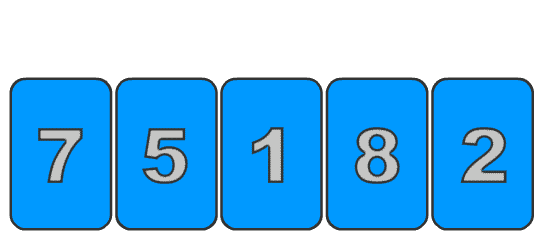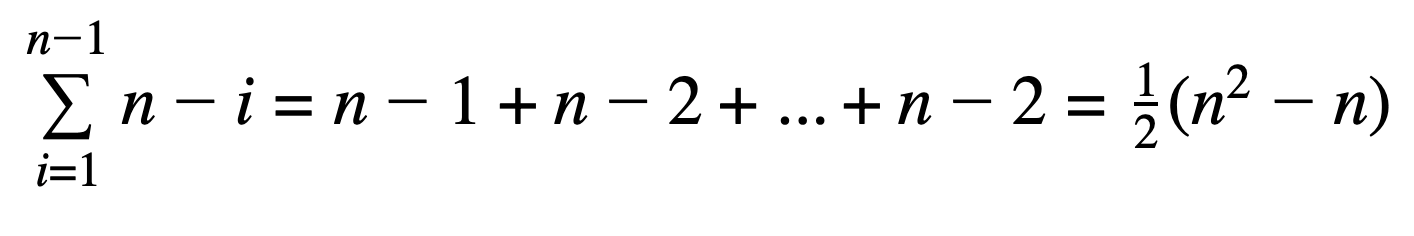# Algorithms: Selection Sort in JavaScript

## How to establish a loop invariant for selection sort and use test-driven development to implement the algorithm

### The Exercise

Recently, I have been brushing up on Cormen’s Introduction to Algorithms book in an effort to become better at interviewing engineering candidates at work. In Chapter 2, which is all about how to design and analyze algorithms, the book presents the following exercise:

Consider sorting n numbers stored in array A by first finding the smallest element of A and exchanging it with the element in A. Then find the second smallest element of A, and exchange it with A. Continue in this manner for the first n-1 elements of A. Write pseudocode for this algorithm, which is known as selection sort. What loop invariant does this algorithm maintain? Why does it need to run for only the first n-1 elements, rather than for all n elements? Give the best-case and worst-case running times of selection sort in Ɵ-notation.

I think this is a great question being that it covers the entire thought process when designing an algorithm. We know the basic idea of how it should work (finding and swapping in the smallest element for each index), but how do we know our implementation is correct? What conditions can we establish to ensure each iteration is performing the correct actions? And lastly, how efficient is the algorithm? What is its run time?

These are all questions we are going to cover for selection sort. I actually just posted a very similar article on insertion sort in JavaScript, which you can find right here:

In that post, I started by introducing the algorithm implementation early on and then covering the correctness and run time analysis. This time, however, I will start by establishing the loop invariant, and then use that to inform my code implementation in JavaScript. That way, we will basically be doing Test-Driven Development (TDD) to implement the algorithm. Once we have done that, we will conclude with the run-time analysis of our implementation.

### The Selection Sort Loop Invariant

As is described in the exercise, selection sort works by iterating over the input, finding the smallest remaining element, and swapping it into the the current index. Pretty straightforward! — but here is a nice gif that I made to help you visualize it :).Selection Sort, Animated

So what would the loop invariant for this algorithm be? As a reminder, the loop invariant is a single condition or set of conditions that the algorithm maintains at the beginning, the end, and during each iteration of its execution. Take a minute or two to think about it before reading further! What properties can we check to ensure our algorithm is running correctly?

Let’s come up with it together now. Based on what we described for how the algorithm works, we know that the smallest remaining element will always be found and sorted first. So after one iteration, the smallest element will be at the 0 index, after two iterations, the two smallest elements will be sorted, and so on and so forth. This property gives us a good condition to check.

The loop invariant for selection sort is that the elements of the newly sorted array up to the current index, `A[0..i]` will contain the `i` smallest elements of our original input, `A[0..n-1]`. They will also be in sorted order.

If this condition holds before, during, and at the end of our algorithm execution, then we can be sure that it is executing correctly.

Here is the code for the loop invariant we just established. It requires the sort-in-progress array, the original input array, and the current index.

We are going to be using this function to help us implement our selection sort function.

NOTE — I am cheating a little bit and using the built-in sort function on the JavaScript array to find the `i` smallest values in the original input. There are other ways to do it, but we are simply going to be using this function essentially as a unit test.

### Implementing Selection Sort

Now that we have our loop invariant function, we can take a test driven development (TDD) approach to implementing the algorithm. Let’s use the same input that is used in the diagram above.

`const input = [7, 5, 1, 8, 2]`

Then, we can create a skeleton function for selection sort and call our loop invariant test function.

We only have to iterate to `nums.length — 1` because, if you think about it, we are constantly swapping the element at each index with the smallest remaining element to the right in the array. By the time we get to the final index, it is guaranteed to be the largest one, so we do not have to process it.

Now, if we run `console.log(selectionSort(input))` we get the following output in our console:

`Error! 7 not one of the 1 smallest elementsError! 7 not one of the 2 smallest elementsError! New Array is not properly sortedError! 5 not one of the 2 smallest elementsError! 7 not one of the 3 smallest elementsError! New Array is not properly sortedError! New Array is not properly sortedError! New Array is not properly sortedError! New Array is not properly sortedError! 8 not one of the 4 smallest elementsError! New Array is not properly sortedError! New Array is not properly sortedError! New Array is not properly sorted[ 7, 5, 1, 8, 2 ]`

This is actually a good thing as it is the start to our TDD approach! We want to implement the algorithm until all of the errors are gone and the final return array is in sorted order.

The inside of the loop is actually pretty simple. We grab the element at the current index, `i`, and then check the remainder of the array for any elements that are smaller than it. Once you have found the smallest element, if it is not already at the current index, swap it with the smallest one.

If you put this code inside of the skeleton code above with the loop invariant checks, you will see that all of our errors are gone and it just outputs the final result!

`[1 2 5 7 8]`

Taking out the loop invariant checks, our final algorithm is:

### Run Time Analysis of Selection Sort

We are going to wrap up this post with a runtime analysis of the selection sort algorithm.

As we learned in the previous section, the selection sort algorithm only needs to run up until the `n-1` element. With that in mind, the outer loop can be represented as a summation from `i=1` to `n-1`. For our inner loop, we only have to iterate over the part of the array that has not yet been sorted. In other words, if we have sorted `i` elements, then we only need to perform `n-i` iterations of the inner loop. That gives us our summation formula:Both the best-case and the worst-case runtime for selection sort

Coincidentally, this is the exact same result that we got for a worst-case insertion sort and it is Ɵ(n²). A huge difference between selection and insertion sort, however, is that selection sort has the same runtime for both its best and worst case. There are no conditions that could possibly short-circuit the inner loop. It always has to loop through the entire array to check that there are no smaller elements remaining. This means that, on average, insertion sort is always going to be a more performant algorithm to use.

Although this means that selection sort is impractical to ever use, as there are more performant algorithms to do the same job, I still found this exercise to be educational and helpful when discussing different algorithmic approaches with candidates and coworkers.